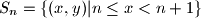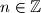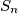### IMO Shortlist 2007 problem C5

Kvaliteta:
Avg: 0,0
Težina:
Avg: 8,0
In the Cartesian coordinate plane define the strips$S_n = \{(x,y)|n\le x < n + 1\}$,$n\in\mathbb{Z}$ and color each strip black or white. Prove that any rectangle which is not a square can be placed in the plane so that its vertices have the same color.

IMO Shortlist 2007 Problem C5 as it appears in the official booklet:In the Cartesian coordinate plane define the strips$S_n = \{(x,y)|n\le x < n + 1\}$ for every integer$n.$ Assume each strip$S_n$ is colored either red or blue, and let$a$ and$b$ be two distinct positive integers. Prove that there exists a rectangle with side length$a$ and$b$ such that its vertices have the same color.

Edited by Orlando Döhring

Author: Radu Gologan and Dan Schwarz, Romania
Izvor: Međunarodna matematička olimpijada, shortlist 2007